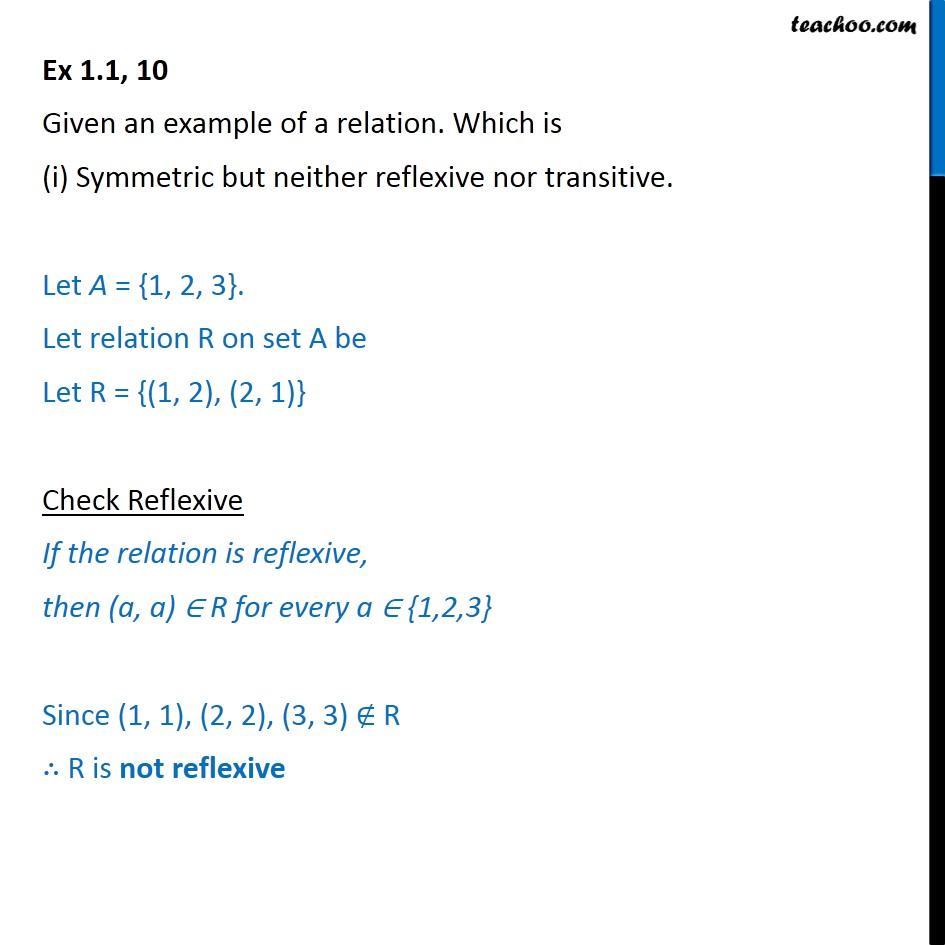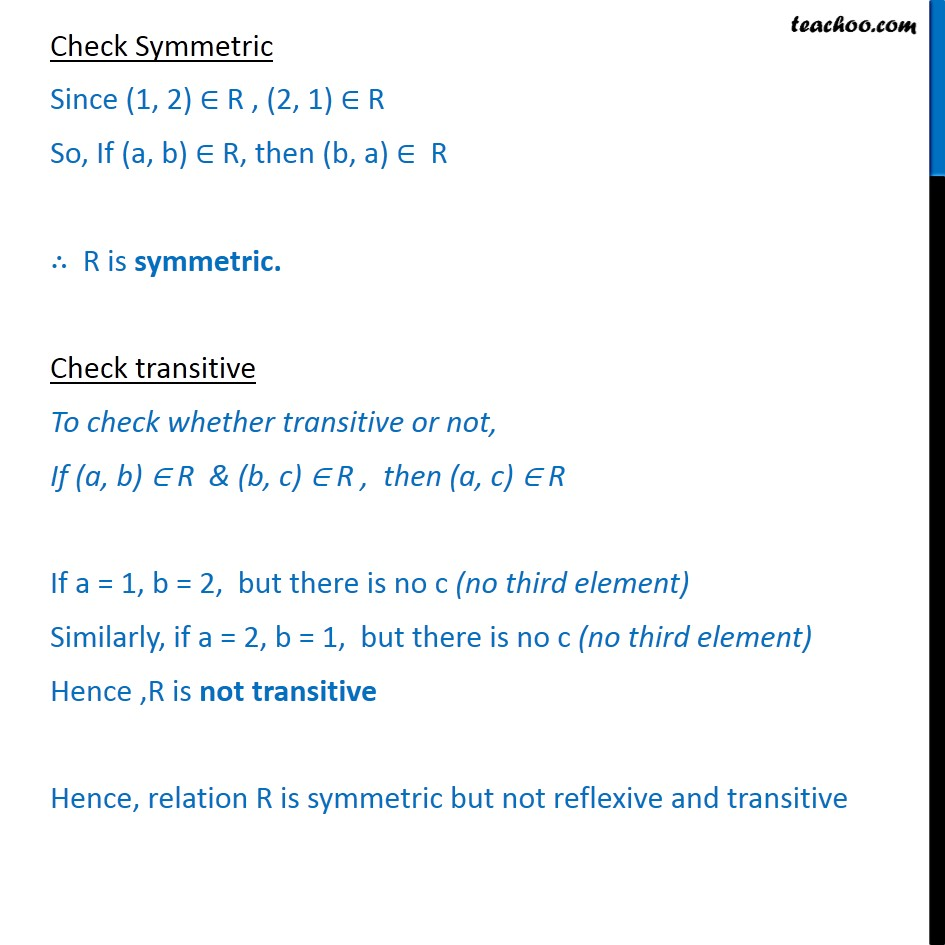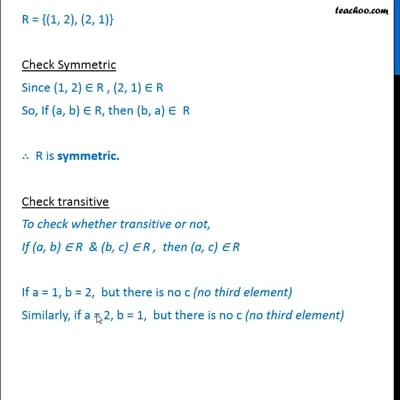To prove relation reflexive, transitive, symmetric and equivalent

Chapter 1 Class 12 Relation and Functions
Concept wiseThis video is only available for Teachoo black users

Introducing your new favourite teacher - Teachoo Black, at only ₹83 per month

### Transcript

Ex 1.1, 10 Given an example of a relation. Which is (i) Symmetric but neither reflexive nor transitive. Let A = {1, 2, 3}. Let relation R on set A be Let R = {(1, 2), (2, 1)} Check Reflexive If the relation is reflexive, then (a, a) R for every a {1,2,3} Since (1, 1), (2, 2), (3, 3) R R is not reflexive Check Symmetric Since (1, 2) R , (2, 1) R So, If (a, b) R, then (b, a) R R is symmetric. Check transitive To check whether transitive or not, If (a, b) R & (b, c) R , then (a, c) R If a = 1, b = 2, but there is no c (no third element) Similarly, if a = 2, b = 1, but there is no c (no third element) Hence ,R is not transitive Hence, relation R is symmetric but not reflexive and transitive